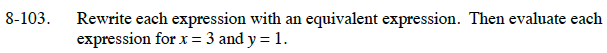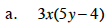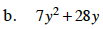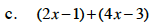Home > CC1 > Chapter 8 > Lesson 8.3.2 > Problem8-103

8-103.
1. Rewrite each expression with an equivalent expression. Then evaluate each expression for x = 3 and y = 1. Homework Help ✎

1. 3x(5y − 4)

2. 7y2 + 28y

3. (2x − 1) + (4x − 3)Multiply what is within the parentheses by what lies on the outside.

15xy − 12x

Substitute in the known values.

15(3)(1) − 12(3)

9Factor out the 7y.First combine like terms to write an equivalent expression.
Then substitute in the known values.

14